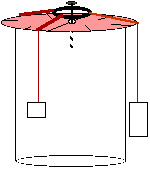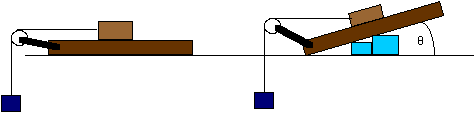# Forces of Nature

#### Lab Assignment: Falling BodiesForces of Nature Lab

# Static Equilibrium

[This is a modification of the AP Physics Lab Force Vector Table. If you intend to submit a lab for AP credit in the Fall Scholars Online Advanced Placement/Honors Physics course, you should the instructions at that site.]

## Goal: To become familiar with force measurements.

This lab, you have several options. You do not need to do both options, only one, but you may wish to experiment with more than one situation.

### Option one: Force table experiments

#### Materials and EquipmentRemember that the setup below is a suggestion; any setup which will produce a force table on which you can read the angles of your as-much-as-possible freely swinging weights will work.

• a metal ring (use frictionless ring from kit if you have it)
• nail
• nylon thread (must be smooth)
• two half-moon protractors made of clear plastic
• stand (for example, a tall thin box, such as an oatmeal carton, with a flat top)
• standard masses (such as nickels, at 5 gr each)

#### Procedure

Assemble the force table as in the diagram. You may need to invert one of the protractors, so be careful when you are reading angles.

1. The biggest source of error in this system will be due to friction where the string touches the table surface, so if you can think of a way to minimize friction there, do so. Physics lab force tables use pulleys for each string; any curved surface will help.
2. Decide which half is the 0°-180° half of the circle, and which is the 180°-360° portion.
3. Tie four nylong strings to the ring. These should be long enough to hang over the edge of your table and allow the attached weights to swing freely.
4. Using three or four weights of differing masses, stabilize the ring around the nail, so that it is centered but not touching the nail.
5. Record your hypothesis: is the sum of the forces in this situation <0, =0, or >0?
6. Record the masses on each string, and the location (0°- 360°) around the table.
7. Draw a force vector diagram of your system in equilibrium.
8. Sum all the horizontal forces.
9. Sum all the vertical forces.
10. What do you expect to get for the sum of all the forces in this situation?
11. Estimate errors for all measurments and discuss their effect on in your measurements.
12. Explain any deviations in experimental data from your calculated data.
13. Repeat this procedure for at least 4 different combinations of masses and positions.

### Option two: Determining the coefficient of friction

#### Materials and Equipment• an elevated surface on which you can slide things without worrying about scratching it. [scrap wood on a table will work--but it shouldn't be too rough].
• a pulley you can clamp to the edge of your surface. If you can't find a pulley, reduce the friction at the edge by using a roll of plastic tubing along the edge.
• several sliders: such as a block of wood, a bar of soap. They should have flat surfaces where they will be in contact with the support surface.
• pins, tape, or other means of fastening string to your slider.
• string
• a set of standard weights (I like using coins; try to weed out any that are too worn). These do not have to be any particular unit, but they should all be the same.
• a bucket, sack, or other means of keeping the weights together.
• blocks or other means of inclining your slide surface
• protractor

#### Procedure

1. Record your hypothesis: do you expect the static friction for a given slider to be a constant, or will it depend on the angle of the sliding surface?
2. Weigh your slide. If you still have your equal arm scale, you can use that. If necessary, just get the weight of your slide in equivalent standard weights (e.g., 95 nickels). If you use a cup to hold your weights, you should weigh it also and include its weight along with your standard weight for total weights below.
3. Fasten the pulley or tubing to your surface edge.
4. Fasten the string to your slide
5. Run the string over the edge of the tubing so that it hangs down; fasten your cup or other weight holder to the end.
6. Start adding weights to the end of the string.
7. Record the total amount of weight required for your slide to start moving.
8. Repeat the above procedure with another slide which has a noticeably smoother or rougher surface.
9. Repeat the above procedure for each slide with the slide surface inclined at approximately 10° and at 15°.
10. Make a table which shows the mass of the slide, the normal force for each trial, and the weight required to overcome static friction. Determine the static friction.
11. Estimate errors for each of your measurements.

Data Reduction